MATLAB Function Referencedde23

Solve delay differential equations (DDEs) with constant delays

Syntax

• ```sol = dde23(ddefun,lags,history,tspan)
sol = dde23(ddefun,lags,history,tspan,options)
```

Arguments

 `ddefun` Function handle that evaluates the right side of the differential equations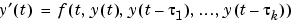. The function must have the form ``````dydt = ddefun(t,y,Z) `````` where `t` corresponds to the current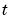, `y` is a column vector that approximates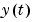, and `Z(:,j)` approximates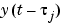for delay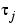= `lags(j)`. The output is a column vector corresponding to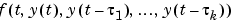. `lags` Vector of constant, positive delays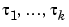. `history` Specify `history` in one of three ways:A function ofsuch that `y = history(t)` returns the solutionfor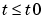as a column vector A constant column vector, if `` is constant The solution `sol` from a previous integration, if this call continues that integration `tspan` Interval of integration as a vector `[t0,tf]` with `t0 < tf`. `options` Optional integration argument. A structure you create using the `ddeset` function. See `ddeset` for details.

Description

```sol = dde23(ddefun,lags,history,tspan) ``` integrates the system of DDEs

•on the interval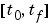, whereare constant, positive delays and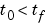.` ddefun` is a function handle. See Function Handles in the MATLAB Programming documentation for more information.

Parameterizing Functions Called by Function Functions, in the MATLAB mathematics documentation, explains how to provide additional parameters to the function `odefun`, if necessary.

`dde23` returns the solution as a structure `sol`. Use the auxiliary function `deval` and the output `sol` to evaluate the solution at specific points `tint` in the interval `tspan = [t0,tf]`.

• ```yint = deval(sol,tint)
```

The structure `sol` returned by `dde23` has the following fields.

 `sol.x` Mesh selected by `dde23` `sol.y` Approximation to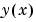at the mesh points in `sol.x`. `sol.yp` Approximation to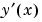at the mesh points in `sol.x` `sol.solver` Solver name, `'dde23'`

```sol = dde23(ddefun,lags,history,tspan,options) ``` solves as above with default integration properties replaced by values in `options`, an argument created with `ddeset`. See `ddeset` and Initial Value Problems for DDEs in the MATLAB documentation for details.

Commonly used options are scalar relative error tolerance `'RelTol'` (`1e-3` by default) and vector of absolute error tolerances `'AbsTol'` (all components are `1e-6` by default).

Use the `'Jumps'` option to solve problems with discontinuities in the history or solution. Set this option to a vector that contains the locations of discontinuities in the solution prior to `t0` (the history) or in coefficients of the equations at known values ofafter `t0`.

Use the `'Events'` option to specify a function that `dde23` calls to find where functions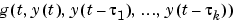vanish. This function must be of the form

• ``````[value,isterminal,direction] = events(t,y,Z)
``````

and contain an event function for each event to be tested. For the `k`th event function in `events`:

• `value(k)` is the value of the `k`th event function.
• `isterminal(k) = 1` if you want the integration to terminate at a zero of this event function and `0` otherwise.
• `direction(k) = 0` if you want `dde23` to compute all zeros of this event function, `+1` if only zeros where the event function increases, and `-1` if only zeros where the event function decreases.

If you specify the `'Events'` option and events are detected, the output structure `sol` also includes fields:

 `sol.xe` Row vector of locations of all events, i.e., times when an event function vanished `sol.ye` Matrix whose columns are the solution values corresponding to times in `sol.xe` `sol.ie` Vector containing indices that specify which event occurred at the corresponding time in `sol.xe`

Examples

This example solves a DDE on the interval [0, 5] with lags 1 and 0.2. The function `ddex1de` computes the delay differential equations, and `ddex1hist` computes the history for `t <= 0`.

 Note    The demo `ddex1` contains the complete code for this example. To see the code in an editor, click the example name, or type `edit ddex1` at the command line. To run the example type `ddex1` at the command line.

• ```sol = dde23(@ddex1de,[1, 0.2],@ddex1hist,[0, 5]);
```

This code evaluates the solution at 100 equally spaced points in the interval `[0,5]`, then plots the result.

• ```tint = linspace(0,5);
yint = deval(sol,tint);
plot(tint,yint);
```

`ddex1` shows how you can code this problem using subfunctions. For more examples see `ddex2`.

Algorithm

`dde23` tracks discontinuities and integrates with the explicit Runge-Kutta (2,3) pair and interpolant of `ode23`. It uses iteration to take steps longer than the lags.

See Also

`ddeget`, `ddeset`, `deval`, `function_handle` (`@`)

References

  Shampine, L.F. and S. Thompson, "Solving DDEs in MATLAB," Applied Numerical Mathematics, Vol. 37, 2001, pp. 441-458.

  Kierzenka, J., L.F. Shampine, and S. Thompson,"Solving Delay Differential Equations with DDE23," available at `www.mathworks.com/dde_tutorial`.

© 1994-2005 The MathWorks, Inc.